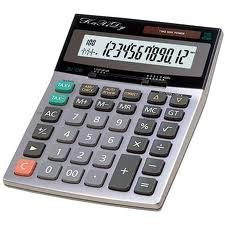Would You Like a Free Expert Consultation?

Not every project goes as well as planned. We would be happy to spend a few minutes answering your questions.

Pro Handyman Blog

What is the Electricity Cost for my Landscape Lights?Landscape lights can really add some pizzazz to a home. Not only can you accent particular features of the landscape and of the home itself, but it also is a good deterrent to prospective burglars. But what are you spending for this feature? It really isn’t hard to figure out.

Here is the formula (and this formula works for other items too):

Watts x hours used/1000 x cost per kilowatt hour (Kwh)

You could go through each light fixture in the yard and add up the wattage of each fixture and plug it into the formula above, but this would take some time. You could also get the total wattage off of the transformer. This wouldn’t be totally accurate though as that number is a maximum, and you shouldn’t exceed 90% of the transformers capacity. So certainly, adding the individual fixtures is more accurate. Just to illustrate the formula and as an acid test, I’m just going to use the 90% figure from the transformer, although I'm not using anywhere near the 90% number.

My current kwh cost from the power company is .13 per kwh ( I say current cost because I know my rate will go up soon). My transformer is 600 watts. If I take 90% of that maximum, I will input 540 watts. If I run my lights 8 hours per night for a 30 day month, I can complete the formula as follows:

540 watts x 240 hours (8 hours per day for 30 days)/1000=129.6 kwh. X .13=\$16.85 per month.

So I pay the power company \$16.85 per month for the privilege of having a landscape light system. Now, this is a fairly large system, so don’t let the numbers scare you. There are much smaller transformers available that can probably handle your needs. My neighbor’s is only 250 watts and he has many slick lights. For example, if we take 90% of a 250 watt transformer you get 225 watts to use. If you are using 13 watt bulbs you could have 17 fixtures on that system. Using the smaller system would cost only  \$7.02 per month (this would assume using the full 90% of the transformers max, which you may not be doing. The point is that the way to get a true cost of your power consumption is to actually count the watts you are using.